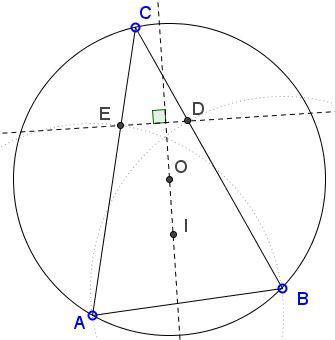# A Property of the Line IOWhat Is This About? A Mathematical Droodle

Explanation### A Property of the Line IO

R. Honsberger in [From Erdos to Kiev, pp. 199-201] treats a problem from Crux Mathematicorum, 1987, 160:

As usual, let I and O be the incenter and circumcenter, respectively, of triangle ABC. Suppose angle C is 30°, and that the side AB is laid off along each of the other two sides to give points D and E so that

EA = AB = BD.Prove that the segment DE is both equal and perpendicular to IO.

Now, I think that the problem is a mixture of two:

1. DE is perpendicular to IO,
2. DE = IO.

The first one is independent of the magnitude of angle C: DE is perpendicular to IO anyway, for any angle C. The second one is the consequence of the angle requirement.

As a preliminary, the construction of points D and E can be repeated for the other two sides AC and BC, giving three lines. Perhaps, curiously, the three are parallel, so that IO can't be perpendicular to them for the reason that angles A, B, C are all 30°.

Below I give solutions posted by Darij Grinberg, one by a participant at one of the AoPS forums, and another by Nathan Bowler. (The published solution appears elsewhere.)

### Solution 1

I shall adopt the convention a > b > c (BC = a, AC = b, AB = c) that is not strictly necessary, but which simplifies the derivation considerably.

It's well known that BX = p - b, where p is the semiperimeter (a + b + c)/2. Thus

(1)
 XA' = BA' - BX = a/2 - (p - b) = a/2 - (a - b + c)/2 = (b - c)/2.

On the other hand, CE = b - c. So that CE = 2·XA' = 2·ID', because ID'A'X is a rectangle (angles at D', A', X are right by the construction.). Similarly, CD = 2·IE'. Furthermore, since ID'||CD and IE'||CE, ∠D'IE' = ∠ECD. Therefore, triangles D'IE' and ECD are similar and 2·D'E' = DE. From here,

∠IE'D' = ∠CDE.

Since the angles at D' and E' are right, ID'E'O is a cyclic quadrilateral with diameter IO. Angles IE'D' and IOD' are subtended by the same chord ID'. They are thus equal. (For a more accurate derivation see, Darij's original.) Wee see that

∠IOD' = ∠CDE.

Also, one pair of corresponding sides is orthogonal (OD'⊥CD) and, since the angles have the same orientation, so must be the other:

DE ⊥ IO.

We also get some extra information. ∠C = ∠ACB = ∠ECD = ∠D'IE'. In ΔD'IE', by the Law of Sines, we have D'E'/sin(∠D'IE') = 2R, where R is the circumradius of ID'E'O, i.e. D'E'/sin(∠D'IE') = IO. With one additional; substitution, we finally get,

DE = 2·IO·sin(∠C),

which, for ∠C = 30°, implies DE = IO.

### Solution 2

In the previous notations,

 ∠XIO + ∠YIO = ∠XIY = ∠180° - ∠C = ∠CDE + ∠CED.

Further, with IO = d,

 sin(∠XIO) = XA'/IO = |b - c|/2d, and sin(∠YIO) = YB'/IO = |a - c|/2d,

which combine into

 sin(∠XIO)/sin(∠YIO) = |b - c|/|a - c| = CE/CD = sin(∠CDE)/sin(∠CED).

It follows that ∠XIO = ∠CDE and ∠YIO = ∠CED. Since IX ⊥ BC and ∠XIO = ∠CDE, DE ⊥ IO. Moreover, from

DE/CE = sin(∠C) / sin(∠CDE),

we obtain

 DE = CE·sin(∠C) / sin(∠CDE) = CE·sin(∠C) / sin(∠XIO) = |b - c|·2d/|b - c|·sin(∠C) = 2d·sin(∠C).

Note: This proof depends on the fact that function fa(t) = sin(t)/sin(a - t) is strictly increasing in the interval (0, a), for 0 < a < π, which can be verified by showing that its derivative is positive on (0, a). But the derivative equals sin(a)/sin2(a - t), which is certainly positive.

### Solution 3

(By Nathan Bowler.) This has a neat proof by transformation geometry.

D is the reflection of A in BI, so DI = AI and

 ∠AID = 2·∠AIB = 2(π - ∠A/2 - ∠B/2) = 2π - (π - ∠C) = π + ∠C.

So letting f be the operation of rotation about I by π + ∠C, D = f(A). Similarly, B = f(E). Let g be the operation of rotation about O by -2∠C. Then g(B) = A, so fgf(E) = D. But fgf rotates the plane by a total angle of (π + ∠C) - 2∠C + (π + ∠C) = 2π, so fgf is a translation by the vector D - E. Let E' = f-1(O), and let D' = E' + D - E = fgf(E') = fg(O) = f(O). Then DE is parallel and equal in length to D'E', which is perpendicular to IO and of length

 D'E' = |2·IO·sin(π + ∠C)| = 2·IO·sin(∠C) = IO

when ∠C = 30°. This also throws some light on the related theorem mentioned elsewhere. Let O' be the reflection of O in CI. Then OO' is at right angles to the bisector CI of ∠ACB, so to the internal bisector of ∠NOM, so it is the external bisector of that angle. So O' is the midpoint of the longer arc MN and

 ∠MIO' = ∠O'IN = ∠MIN/2 = (2π - 2∠C)/2 = π - ∠C.

So ∠O'IM = ∠NIO' = π + ∠C, and so M and N are the reflections of D' and E' in CI. Thus MN is equal and antiparallel to D'E', which is equal and parallel to DE. Thus MN, NO and OM are antiparallel to ED, DC and CE respectively, so MNO and EDC are similar. As also MN = ED, they are congruent.

### References

1. R. Honsberger, From Erdös To Kiev, MAA, 1996.